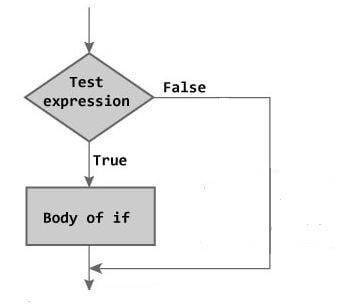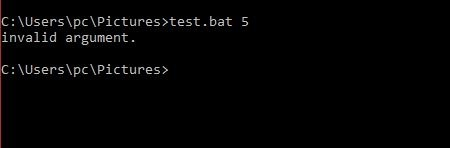# Batch file if else statementsThe batch script supports the conditional statements like if, if else ..etc. In this article, I will discuss how you can use the if and else in the batch file.

You can see this article, Batch file commands

### Batch file if statement

The if statement is one of the selection statements. It uses to select statements depending on the value of a controlling expression.

#### Syntax,

In the above scenario, the statement will only be executed if the controlling expression is non-zero.Let see  an example,

The below script displays the message according to the argument enter by the user.

When User Enter 4:When User Enter 6:When User Enter anything except 4 and 6:### Batch file if else statement

if else is a selection statement that used to select statements depending on the value of a controlling expression.

#### Syntax:

In the above scenario, the statement1 will only be executed if the expression is non-zero. if the expression is zero, then statement2 will be executed.Let see an example,

The below program check even number and odd number. If a number is divided by 2, it means it is an even number. If the number is not divided by 2, then it is an odd number.

### How the above program works:

1 Case: When you entered even number:

We know that the modular division of an even number by 2 is 0. So expression (num%% 2) return 0, as we know expression ( 0 == 0) return 1. Now controlling the expression of if statement is non-zero then body associated with if statement will execute.2 Case: When you entered an odd number:

For odd number expression (num %% 2) return 1, so expression ( 1== 0) return 0. Now controlling the expression of if statement is zero then body associated with if statement will skip.